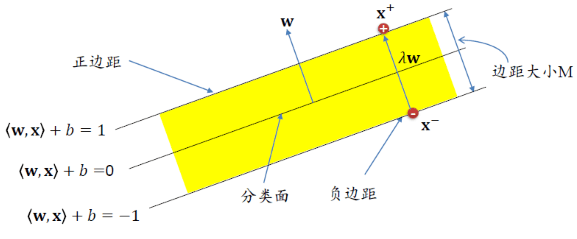0%

• 线性可分SVM
• SVM对偶问题

## 线性可分SVM

PS: 支持向量机的输入和感知机是一样的。

PS: 原来的$$y_i({\bf w^T x_i} + b) >0$$的条件限制不如新的条件，因此略去了。\begin{align} &{\bf w^T x^+} + b = +1 & \\&{\bf w^T x^-} + b =-1 & \\联立两式：&{\bf w^T (x^+ - x^-) } = 2& \\ 因为：& {\bf x^+ = x^- + \lambda w } &\Rightarrow \lambda = \frac{2}{\bf w^Tw} \\间距和为：& |\!|\bf{x^+ - x^-}|\!| = |\!|\lambda w|\!|= \frac{2}{\bf w^Tw} |\!|w|\!| = \frac{2}{|\!|w|\!|} \\然后最大化&\frac{2}{|\!|w|\!|}和最小化 \frac{1}{2}{\bf w^Tw}等价 \end{align} 但其实限制条件是从上面这样缩放而来的，因此本文关于原问题的基本是按照林轩田老师和李航老师的思路写的。

## SVM对偶问题

1. 对偶问题往往更容易求解
2. 自然引入核函数，进而推广到非线性分类问题

$${\bf z} = \Phi({\bf x})$$, 则SVM 原始问题写为： \begin{align*} \min_{\bf w,b}\hspace{3ex} & \frac{1}{2} {\bf w^Tw}\\ {\rm s.t.}\hspace{3ex} & y_i({\bf w^T \Phi({x})} + b) \ge 1, \hspace{3ex}i=1,2,\cdots ,n \end{align*} x经过非线性特征转换以后，映射到新空间里得到的z通常维度比较高。

### 拉格朗日乘子法

• 当g不满足约束条件的时候（$$g_i(x) \gt 0$$），我们内层优化取max，因此$$\alpha_i = \infty \Rightarrow \alpha_ig_i({\bf x}) = \infty$$
• 当g满足约束条件的时候（$$g_i(x) \le 0$$），我们内层优化取max，因此$$\alpha_i =0 \Rightarrow \alpha_ig_i({\bf x}) = 0$$
• 当h不满足约束条件的时候（$$h_j(x) \ne0$$），同理可以取$$\beta_j= \rm sign(h_j({\bf x})) \infty \Rightarrow \beta_jh_j({\bf x}) = \infty$$
• 当h满足约束条件的时候（$$h_j(x) =0$$），同理可以取$$\beta_jh_j({\bf x}) =0$$

• 主问题可行： $$g_i({\bf x}) \le 0, h_i({\bf x}) = 0$$
• 对偶问题可行： $$\alpha_i \ge 0$$
• 互补松弛: $$\alpha_i g_i({\bf x}) = 0$$

### 拉格朗日对偶问题

2-2只是交换了max-min的顺序，得到的就是拉格朗日对偶问题

### SVM 对偶问题推导

$\sum_{i=1}^n \alpha_iy_i = 0 \tag{2-6}$

2-7就是我们的SVM对偶问题。需要满足的KKT条件为：

• 主问题可行： $$1 - y_i({\bf w^T x_i} + b) \le0$$
• 对偶问题可行：$$\alpha_i \ge 0$$
• 互补松弛: $$\alpha_i \left( 1 - y_i({\bf w^T x_i} + b) \right) = 0$$
• 2-5和2-6的条件: $${\bf w} = \sum_{i=1}^n \alpha_iy_i{\bf x_i} ; \sum_{i=1}^n \alpha_iy_i = 0$$

PS: 周志华老师的KKT条件中没有2-5和2-6两式，而李航老师和林轩田老师的都有。 笔者认为也应该加上: )

### 求解对偶问题

2-7所表示的SVM对偶问题仍可以用二次规划算法来求解，但是问题规模正比于训练样本数，在实际任务中开销很大。往往采用更高效的算法如SMO或者 Pegasos ，这将在最后介绍。

• $${\bf w} = \sum_{i=1}^n \alpha_iy_i{\bf x_i}$$
• 选取一个$$\alpha_i \gt 0$$ 可得$$y_i({\bf w^T x_i} + b) = 1 \Rightarrow {\bf w^T x_i} + b= y_i \Rightarrow b = y_i - {\bf w^T x_i}$$

1. 只需要优化$$\alpha$$而不是b和w，降低了算法的时间复杂度
2. 通过查看$$a_i > 0$$ 便可找出支持向量

## 参考资料

• 机器学习技法 - 林轩田
• 《统计学习方法》 - 李航
• 《机器学习》 - 周志华
• 《从零构建支持向量机(SVM)》 - 张皓